• import matplotlib.pyplot as plt x = [1, 2, 3, 4] y = [1, 4, 9, 6] labels = ['Frogs', 'Hogs', 'Bogs', 'Slogs'] plt.plot(x, y) # You can specify a rotation for the tick labels in degrees or with ...
import matplotlib.pyplot as plt

x = [1, 2, 3, 4]
y = [1, 4, 9, 6]
labels = ['Frogs', 'Hogs', 'Bogs', 'Slogs']

plt.plot(x, y)
# You can specify a rotation for the tick labels in degrees or with keywords.
plt.xticks(x, labels, rotation='vertical')
# Pad margins so that markers don't get clipped by the axes
plt.margins(0.2)
# Tweak spacing to prevent clipping of tick-labels
plt.subplots_adjust(bottom=0.15)
plt.show()

通过 plt.subplots_adjust(bottom=0.15)，来调整 图表 的上下左右。

https://matplotlib.org/api/_as_gen/matplotlib.pyplot.subplots_adjust.html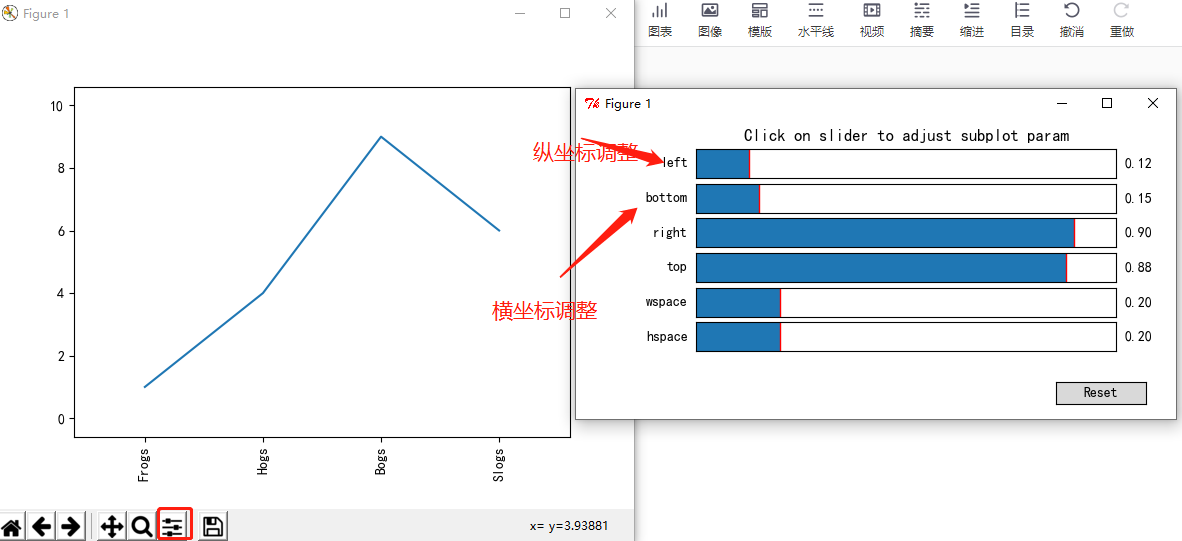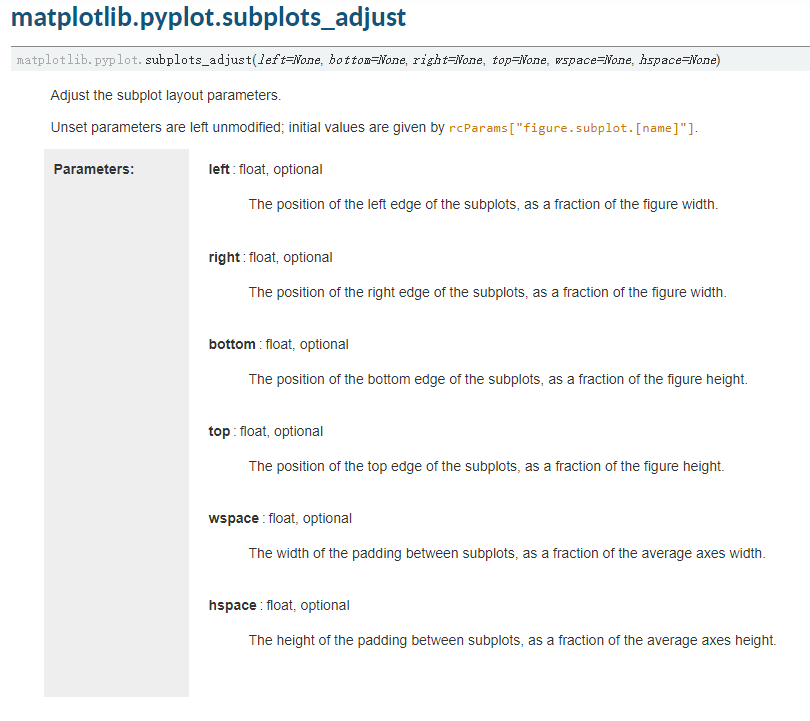参考：

1. https://blog.csdn.net/weixin_35834894/article/details/98874947


展开全文python
• 正向显示纵轴文字,使用rotation参数 可以在plt.ylabel()中增加rotation=0参数,是文字正向显示如 ax2 = plt.subplot(122) ax2.scatter(X[:,1],Y[:,0], c = "g") ...直接看效果,左边加,右边加上 ...
正向显示纵轴文字,使用rotation参数
可以在plt.ylabel()中增加rotation=0参数,是文字正向显示如
ax2 = plt.subplot(122)
ax2.scatter(X[:,1],Y[:,0], c = "g")
plt.xlabel("x2")
plt.ylabel("y",rotation = 0)
plt.show()

直接看效果,左边不加,右边加上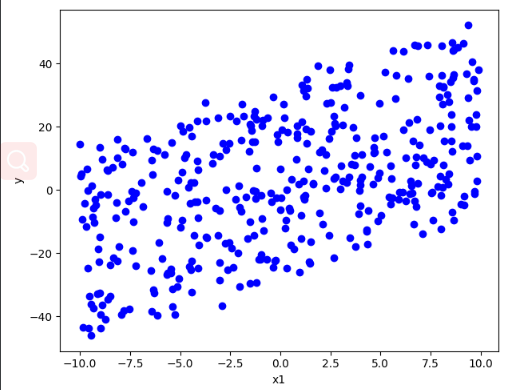展开全文python
• matplotlib画二维图像时，默认情况下的横坐标和纵坐标显示的值有时达到自己的需求，需要借助xticks()和yticks()分别对横坐标x-axis和纵坐标y-axis进行设置。 import numpy as np import matplotlib.pyplot as ...
用matplotlib画二维图像时，默认情况下的横坐标和纵坐标显示的值有时达不到自己的需求，需要借助xticks()和yticks()分别对横坐标x-axis和纵坐标y-axis进行设置。

import numpy as np
import matplotlib.pyplot as plt
x = range(1,13,1)
y = range(1,13,1)
plt.plot(x,y)
plt.show()

x坐标和y坐标都表示1到12的整数，不进行坐标设置时，执行效果为：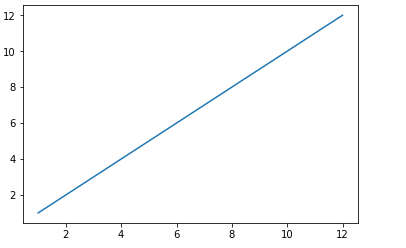此时的x轴和y轴都是只显示偶数，其它的奇数未显示，这样在展示实验效果或放入文章中都会影响其可读性。

为了设置坐标轴的值，增加其可读性，有多种方法。这里介绍的是matplotlib的函数xticks()和yticks()。

参考文档：xticks()函数介绍      yticks()函数介绍

xticks()中有3个参数：

xticks(locs, [labels], **kwargs)  # Set locations and labels


locs参数为数组参数(array_like, optional)，表示x-axis的刻度线显示标注的地方，即ticks放置的地方，上述例子中，如果希望显示1到12所有的整数，就可以将locs参数设置为range(1,13,1)，第二个参数也为数组参数(array_like, optional)，可以不添加该参数，表示在locs数组表示的位置添加的标签，labels不赋值，在这些位置添加的数值即为locs数组中的数。如下图

import numpy as np
import matplotlib.pyplot as plt
x = range(1,13,1)
y = range(1,13,1)
plt.plot(x,y)
plt.xticks(x)
plt.show()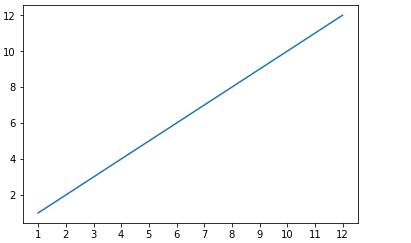xticks()函数中，locs参数为数组x，即1到12所有的整数， 即画出的图像会在这12个位置画出ticks，即上图中的刻度线。

当赋予labels的值为空时，则在locs决定的位置上虽然会画出ticks，但不会显示任何值。

import numpy as np
import matplotlib.pyplot as plt
x = range(1,13,1)
y = range(1,13,1)
plt.plot(x,y)
plt.xticks(x,())
plt.show()

其效果为：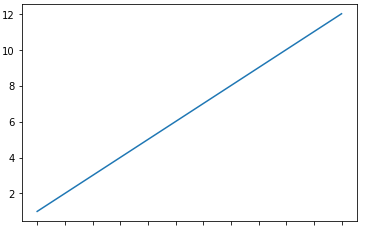该例子中，会明显看到locs和labels的关系，locs表示位置，labels决定这些位置上的标签，labels的默认值为和locs相同。

所以，对于labels参数，我们可以赋予其任意其它的值，如人名，月份等等。

import numpy as np
import matplotlib.pyplot as plt
x = range(1,13,1)
y = range(1,13,1)
plt.plot(x,y)
plt.xticks(x, ('Tom','Dick','Harry','Sally','Sue','Lily','Ava','Isla','Rose','Jack','Leo','Charlie'))
plt.show()

在每个标签会依次显示labels中的人名：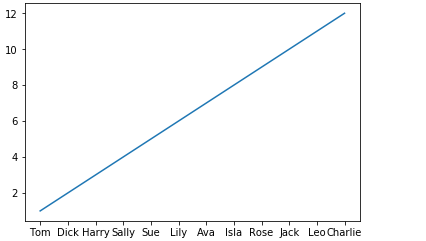还可以显示月份：

import numpy as np
import matplotlib.pyplot as plt
import calendar
x = range(1,13,1)
y = range(1,13,1)
plt.plot(x,y)
plt.xticks(x, calendar.month_name[1:13],color='blue',rotation=60)
plt.show()

显示效果为：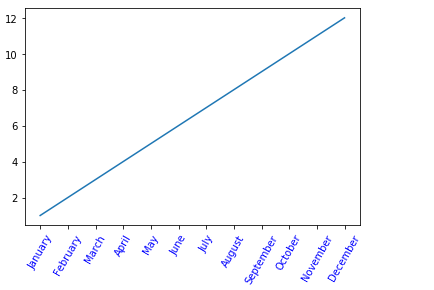这里添加了 calendar 模块，用于显示月份的名称。calendar.month_name[1:13]即1月份到12月份每个月份的名称的数组。后面的参数color='blue'表示将标签颜色置为蓝色，rotation表示标签逆时针旋转60度。

通过上个示例，可看出第3个参数关键字参数**kwargs用于控制labels，具体可通过Text属性中的定义，添加到该参数中，关于其定义可参考在 Text 查询。

另外，通过第1个参数locs可以看出，xticks()函数还可以用来设置使x轴上ticks隐藏，即将空数组赋予它，则没有tick会显示在x轴上，此处参考：x轴数值隐藏。

import numpy as np
import matplotlib.pyplot as plt
import calendar
x = range(1,13,1)
y = range(1,13,1)
plt.plot(x,y)
plt.xticks([])
plt.show()

可看出x轴上没有tick显示：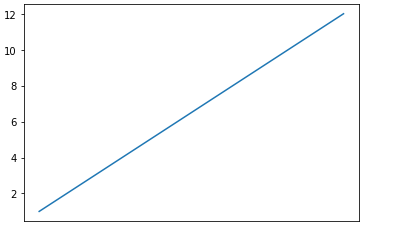同理，对于yticks()函数定义和xticks()函数定义完全相同。对于第一个例子，如果希望在y轴上的刻度线也显示1到12所有的整数，则将lens(1,13,1)赋予yticks()的locs参数即可：

import numpy as np
import matplotlib.pyplot as plt
import calendar
x = range(1,13,1)
y = range(1,13,1)
plt.plot(x,y)
plt.xticks(x)
plt.yticks(y)
plt.show()

其效果为：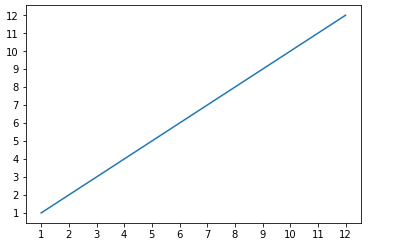综上，可以设计一个x轴为月份，y为星期的图像：

import numpy as np
import matplotlib.pyplot as plt
import calendar
from datetime import *
x = range(1,13,1)
y = range(1,13,1)
plt.plot(x,y)
plt.xticks(x, calendar.month_name[1:13],color='blue',rotation=60)
today = datetime(2018, 9, 10)
a=[]
for i in range(12):
a.append(calendar.day_name[today.weekday()+(i%7)])
plt.yticks(y,a,color='red')
plt.show()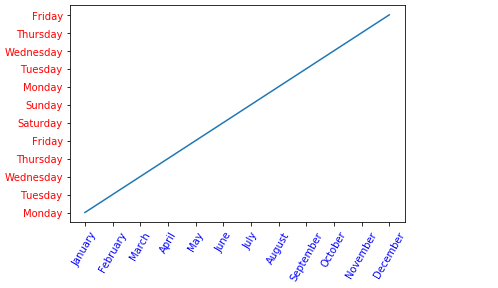对应一月份选择星期一，二月份选择星期二，往后依次类推，直至将12个月安排完。 
展开全文pytho xticks ticks
• 成功解决matplotlib绘图的时候横坐标或纵坐标文本显示不全/显示一半/显示不完整的问题 目录 解决问题 解决思路 解决方法 解决问题 成功解决matplotlib绘图的时候横坐标或纵坐标文本显示不...
成功解决matplotlib绘图的时候横坐标或纵坐标文本显示不全/显示一半/显示不完整的问题

目录

解决问题

解决思路

解决方法

解决问题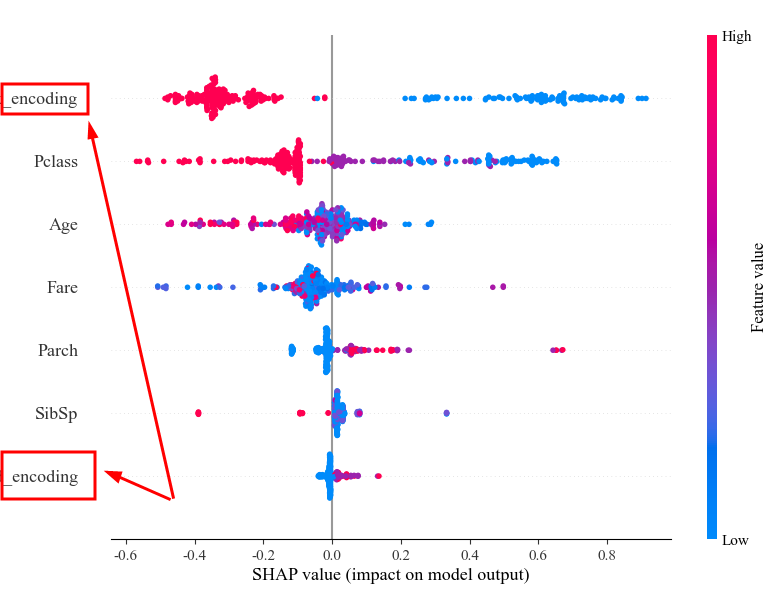成功解决matplotlib绘图的时候横坐标或纵坐标文本显示不全/显示一半/显示不完整的问题

解决思路

横坐标或纵坐标文本显示不全/显示一半/显示不完整，Adjust the padding between and around subplots，需要调整subplots之间和周围的填充。

解决方法

在plt.show()之前，添加下边一行即可！

plt.tight_layout()
plt.show()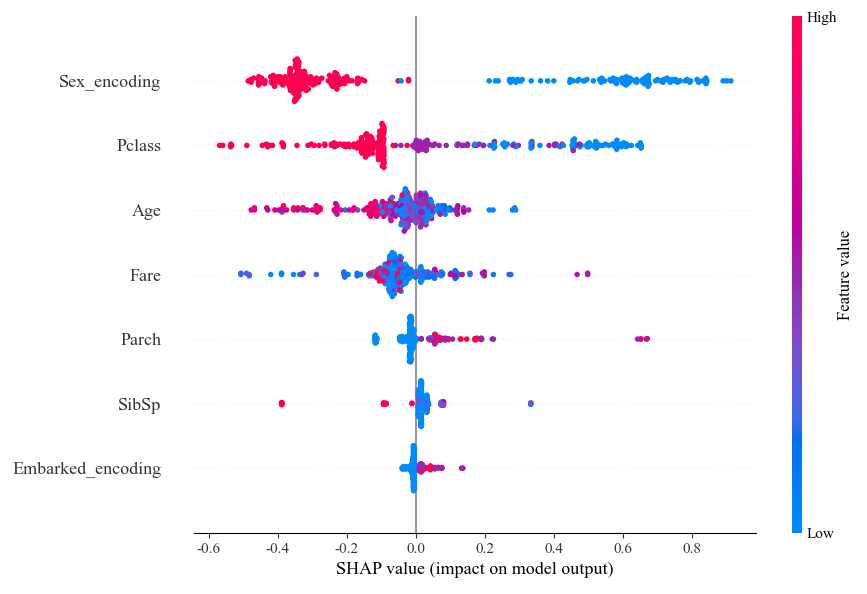哈哈，大功告成！


展开全文• python_matplotlib改改变变横横坐坐标标和和纵纵坐坐标标上上的的刻刻度度(ticks)方方式式 这篇文章...用matplotlib画二维图像时默认情况下的横坐标和纵坐标显示的值有时达到自己的需求需要借 xticks)和yticks )分
• matplotlib画二维图像时，默认情况下的横坐标和纵坐标显示的值有时达到自己的需求，需要借助xticks()和yticks()分别对横坐标x-axis和纵坐标y-axis进行设置。 import numpy as np import matplotlib.pyplot as ...
• matplotlib画二维图像时，默认情况下的横坐标和纵坐标显示的值有时达到自己的需求，需要借助xticks()和yticks()分别对横坐标x-axis和纵坐标y-axis进行设置。import numpy as npimport matplotlib.pyplot as pltx...
• matplotlib画二维图像时，默认情况下的横坐标和纵坐标显示的值有时达到自己的需求，需要借助xticks()和yticks()分别对横坐标x-axis和纵坐标y-axis进行设置。import numpy as npimport matplotlib.pyplot as pltx...
• matplotlib画二维图像时，默认情况下的横坐标和纵坐标显示的值有时达到自己的需求，需要借助xticks()和yticks()分别对横坐标x-axis和纵坐标y-axis进行设置。import numpy as npimport matplotlib.pyplot as pltx...
• 背景现有一个名为df的DataFrame如下通过如下代码ax 绘制出需求图例显示出中文横坐标标签中WV-IR11为下标横纵坐标末端有箭头纵坐标刻度用百分比表示绘制x=-2的虚线图例的外边框不显示实现import matplotlib.pyplot as...
• 本文想说的是折线点的地方显示数据纵坐标数据 利用：plt.text() 方法 废话多说，直接上代码： # encoding=utf-8 import matplotlib.pyplot as plt # 月份 x1 = ['2017-01', '2017-02', '2017-03', '2017-04...python 折线图
• 显示中文、下标、坐标末端箭头；纵坐标刻度用百分比表示；绘制垂直于坐标轴的线；图例的外边框不显示python
• 我的想法是按照铺店率从大到小排序作为横坐标，然后纵坐标是销售量。那么 常理先搞定头文件 import matplotlib.pyplot as plt import matplotlib as mpl import pandas as pd 用pandas读取excel中文也可以，之前...
• matplotlib画二维图像时，默认情况下的横坐标和纵坐标显示的值有时达到自己的需求，需要借助xticks()和yticks()分别对横坐标x-axis和纵坐标y-axis进行设置。import numpy as npimport matplotlib.pyplot as plt...
• matplotlib画二维图像时，默认情况下的横坐标和纵坐标显示的值有时达到自己的需求，需要借助xticks()和yticks()分别对横坐标x-axis和纵坐标y-axis进行设置。importnumpyasnpimportmatplotlib.pyplotaspltx=range...
• 用plot画二维图像时，默认情况下的横坐标和纵坐标显示的值有时达到自己的需求，需要借助xticks()和yticks()分别对横坐标x-axis和纵坐标y-axis进行设置。import numpy as npimport matplotlib.pyplot as pltx = ...
• matplotlib画二维图像时，默认情况下的横坐标和纵坐标显示的值有时达到自己的需求，需要借助xticks()和yticks()分别对横坐标x-axis和纵坐标y-axis进行设置。 import numpy as np import matplotlib.pyplot as ...python 数据分析 可视化 java vue
• matplotlib画二维图像时，默认情况下的横坐标和纵坐标显示的值有时达到自己的需求，需要借助xticks()和yticks()分别对横坐标x-axis和纵坐标y-axis进行设置。import numpy as npimport matplotlib.pyplot as plt...
• ,y='hwy'# 纵坐标，公路里程 ,hue='cyl'#分类，气缸数量 ,data=df_select #,height=8# 图像的高度 ,aspect=1.6#图像的纵横比 ,legend=False ,palette='tab10'#色板 ,scatter_kws=dict(s=60,linewidth...python
• ` from matplotlib import pyplot as plt from matplotlib import font_manager x = ('碳酸饮料', '绿茶', '矿泉水', '果汁', '其他') ...fonts = font_manager.FontProperties...为什么图片纵坐标显示字体全？python
• ## python 常用包总结

万次阅读 2017-10-07 13:13:04
1、 Import 函数 from 库，往后可以直接使用 函数 import库，要使用函数则需 库....3、 用图形表示回归效果可以采用横坐标为实际值，纵坐标为预测值（采用横坐标的标定作为标定）则预测点越集中在y=x坐标
• 1、 Import 函数 from 库，往后可以直接使用 函数 import库，要使用函数则需 库....3、 用图形表示回归效果可以采用横坐标为实际值，纵坐标为预测值（采用横坐标的标定作为标定）则预测点越集中在y=x坐标
• 2、 %matplotlib inline是jupyter notebook里的命令, 意思是将那些用matplotlib绘制的图显示在页面里而不是弹出一个窗口3、 用图形表示回归效果可以采用横坐标为实际值，纵坐标为预测值(采用横坐标的标定作为标定)则...
• 2、 %matplotlib inline是jupyter notebook里的命令, 意思是将那些用matplotlib绘制的图显示在页面里而不是弹出一个窗口3、 用图形表示回归效果可以采用横坐标为实际值，纵坐标为预测值(采用横坐标的标定作为标定)则...
• plt.plot(range(1, len(ppn.errors_) + 1), ppn.errors_, marker='o') # 横坐标从1到len(errors_),纵坐标为errors_， plt.xlabel('Epochs') plt.ylabel('Number of misclassifications') plt.show() plot...机器学习